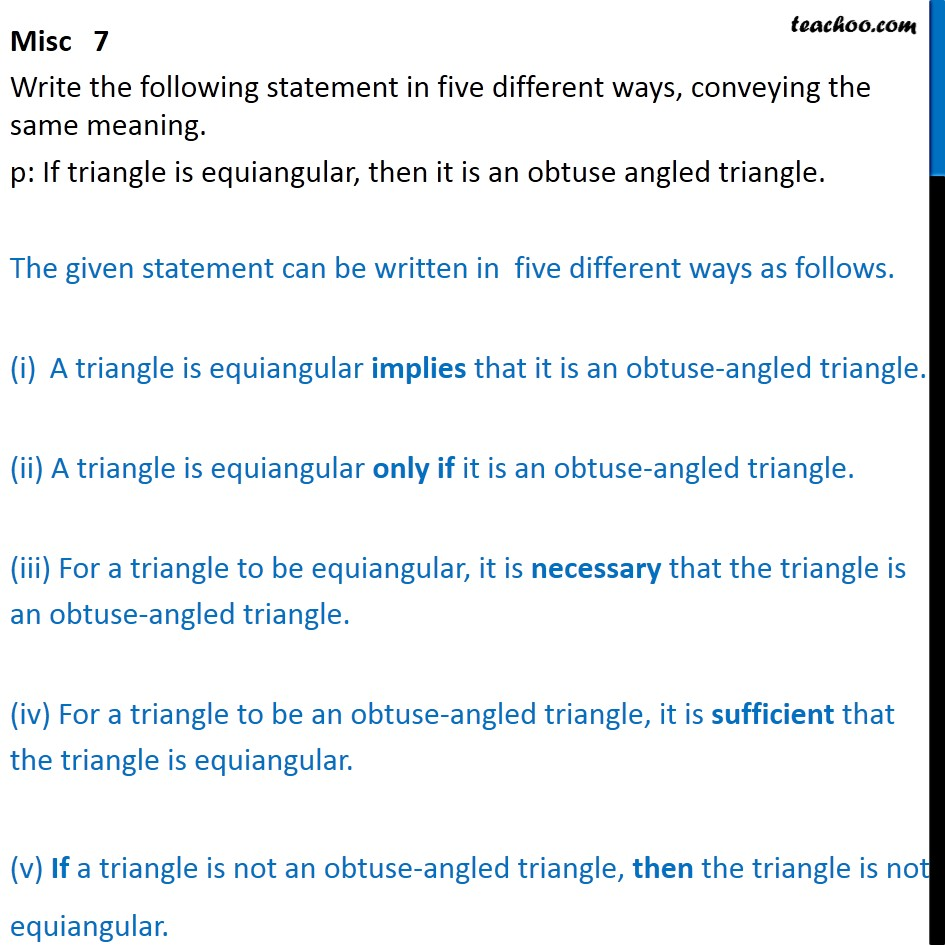Miscellaneous

Mathematical Reasoning
Serial order wiseLearn in your speed, with individual attention - Teachoo Maths 1-on-1 Class

### Transcript

Misc 7 Write the following statement in five different ways, conveying the same meaning. p: If triangle is equiangular, then it is an obtuse angled triangle. The given statement can be written in five different ways as follows. A triangle is equiangular implies that it is an obtuse-angled triangle. (ii) A triangle is equiangular only if it is an obtuse-angled triangle. (iii) For a triangle to be equiangular, it is necessary that the triangle is an obtuse-angled triangle. (iv) For a triangle to be an obtuse-angled triangle, it is sufficient that the triangle is equiangular. (v) If a triangle is not an obtuse-angled triangle, then the triangle is not equiangular.